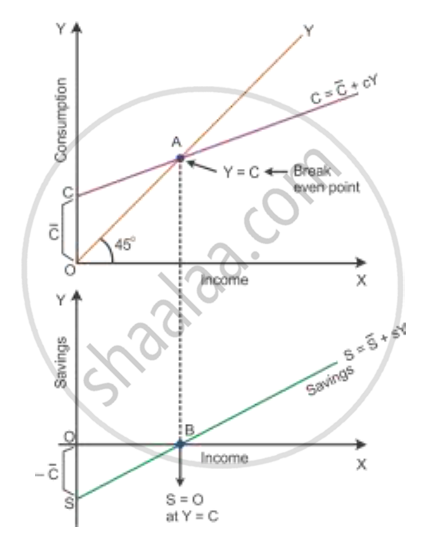# Given Saving Curve, Derive Consumption Curve and State the Steps in Doing So. Use Diagram. - Economics

Short Note

Given saving curve, derive consumption curve and state the steps in doing so. Use diagram.

Outline the steps required to be taken in deriving the Consumption Curve from the given Saving Curve. Use Diagram.

#### Solution

Steps taken for derivation of consumption curve are:
(i) At zero level of income, the savings are O\overline{S} which is the amount of autonomous consumption at Y= 0. So, -O\overline{S} = O\overline{C}. Hence, the consumption will start from the point \overline{C}.
(ii) We draw a 45° line passing through the origin which shows that C = Y. This is the income line.
(iii) Now, we draw a vertical line from the point E, where saving is zero. At zero level of saving, C = Y. So, B is the break-even point.
(iv) The consumption curve is derived meeting C and B and extending it forward.

Derivation of the consumption curve from the saving curve:-

Given the SS curve, let us consider OS = OC. At Point B, draw a perpendicular 45° line towards Point A. Points C and A are joined to produce a straight line upward sloping consumption curve CC.Concept: Supply Curve and Schedule
Is there an error in this question or solution?
2015-2016 (March) All India Set 1

Share